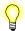# atan2(), atan2f()

Compute the arctangent, determining the quadrant

## Synopsis:

```#include <math.h>

double atan2( double y,
double x );

float atan2f( float y,
float x );```

## Arguments:

x, y
The value (y/x) for which you want to find the angle.

## Library:

libm

Use the -l m option to qcc to link against this library.

## Description:

These functions compute the value of the arctangent (in radians) of y/x, using the signs of both arguments to determine the quadrant of the return value. A domain error occurs if both arguments are zero.

## Returns:

The arctangent of y/x, in the range (-π, π).If an error occurs, these functions return 0, but this is also a valid mathematical result. If you want to check for errors, set errno to 0, call the function, and then check errno again. These functions don't change errno if no errors occurred.

## Examples:

```#include <stdio.h>
#include <math.h>
#include <stdlib.h>

int main( void )
{
printf( "%f\n", atan2( .5, 1. ) );

return EXIT_SUCCESS;
}```

produces the output:

`0.463648`

## Classification:

Safety:
Cancellation point No
Interrupt handler No
Signal handler No
Thread Yes